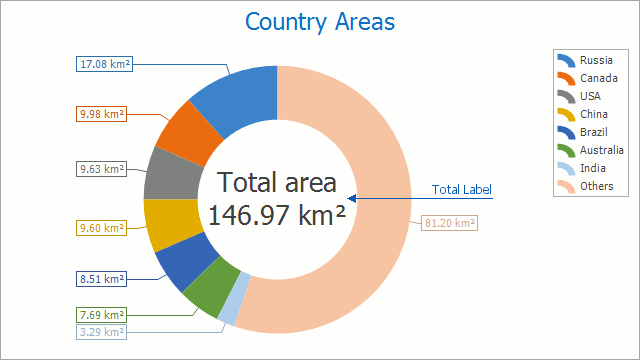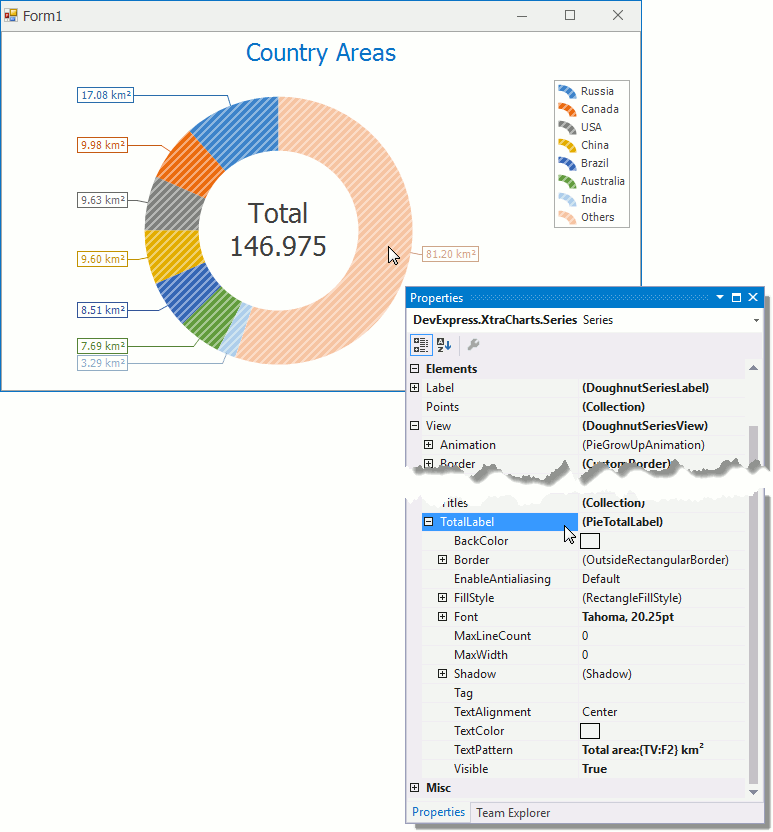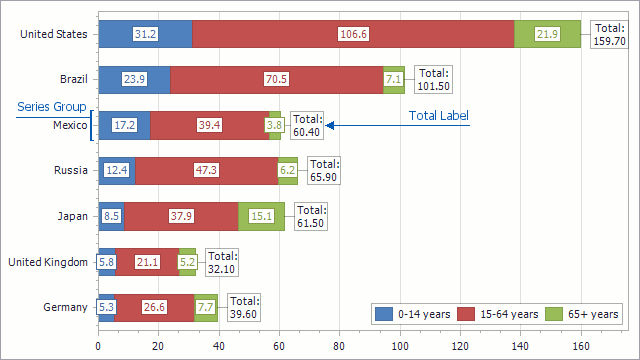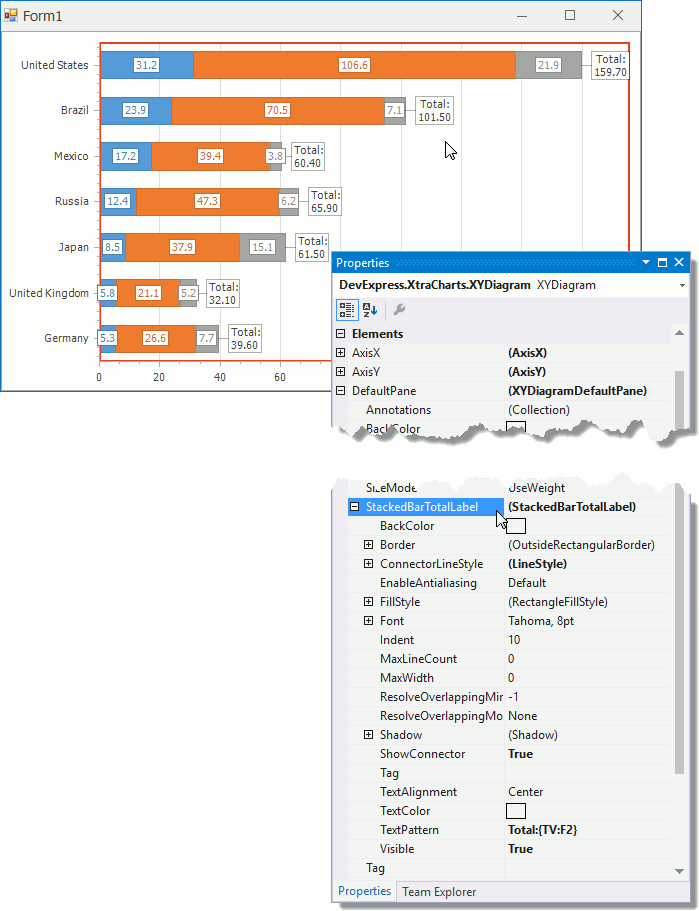20.1
19.2
19.1
18.2
18.1
17.2

# Total Labels

Total labels display several series points' total value. A Series View type defines which series points are used to calculate the total value. Stacked Bar Total Labels show Stacked Bar series groups' total values. The Pie Total Label displays Pie (Doughnut) series points' total value.

The following sections describe the types of Total Labels:

## Pie Total Label

The Pie Total Label displays the total value of all Pie (Doughnut) series' points.Follow the steps below to access the Pie Total Label's settings at design time.

1. Select the Pie series whose Total Label you want to configure.
2. In the Properties window, expand the SeriesBase.View property.
3. Expand the view's PieSeriesView.TotalLabel property.
4. Configure the total label's parameters to meet your requirements.The same customization can be performed at the runtime. The example below demonstrates this.

Use the PieSeriesView.TotalLabel property to access settings that configure a Pie (Doughnut) series' Total Label.

##### NOTE

A complete sample project is available at https://github.com/DevExpress-Examples/how-to-show-a-pie-series-total-label-t556267

``````PieTotalLabel totalLabel = ((DoughnutSeriesView)pieChart.Series["Country Areas"].View).TotalLabel;
totalLabel.Visible = true;
totalLabel.TextPattern = "Total area\n{TV:F2} km\xB2";
``````

## Stacked Bar Total Labels

Stacked Bar Total Labels show Stacked Bar series groups' total values.Follow the steps below to access the Stacked Bar Total Labels' settings at design time.

1. Select a Pane on which Stacked Bar Series that Total Labels should accompany are plotted.
2. In the Properties window, expand the XYDiagramPaneBase.StackedBarTotalLabel property.

##### NOTE

Total Labels' settings are not shared between panes, and you can configure them individually.

3. Configure the Total Label's parameters to meet your requirements.The same customization can be performed at the runtime. The example below demonstrates this.

The XYDiagramPaneBase.StackedBarTotalLabel property allows you to configure total labels of stacked bars that are in the pane. Note that Total labels' settings are not shared between Panes. Thus, you can configure Total Labels that are on various panes differently.

##### NOTE

A complete sample project is available at https://github.com/DevExpress-Examples/how-to-show-stacked-bars-total-labels-at-runtime-t556236

``````StackedBarTotalLabel totalLabel = ((XYDiagram)cartesianChart.Diagram).DefaultPane.StackedBarTotalLabel;
totalLabel.Visible = true;
totalLabel.ShowConnector = true;
totalLabel.TextPattern = "Total:\n{TV:F2}";
``````